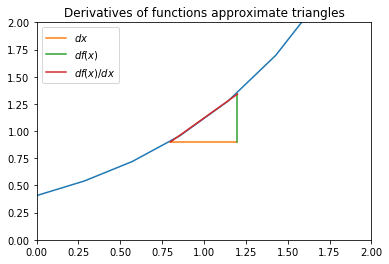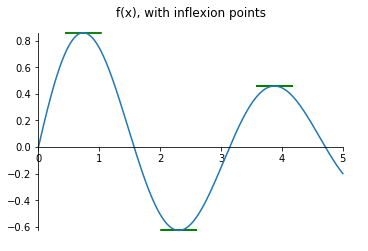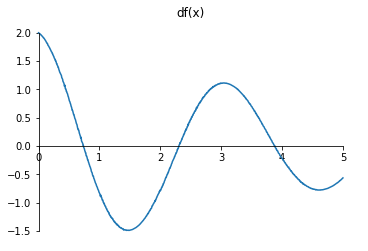Notebook

# Maths

In :
% run maths_functions.py


## Differentiation

A derivative is the rate of change of a certain function with respect to a certain variable. For example for a moving object such as a car, the rate of change distance with comparison to time is denoted as $\cfrac{d s(t)}{dt} = v$, where v is the velocity. A derivative can also be denoted with a quotation mark next to the variable.

In the simple case where the rate of change is constant, v is the division of the total distance by the total time. However, in reality that is usually not the case. That is why the derivative varies with each point at which it is taken. To compute it, the dividend and the divisor (in this example ds and dt) are assumed to be infinitesimally small changes in the arguments.

$v = \cfrac{\triangle{s}}{\triangle{t}} = \cfrac{s(t+\triangle{t}) - s(t)}{t+\triangle{t} - t}$, where $\triangle{t} \to 0$

With this definition it becomes clear that for a function f(x), the derivative at a point, is the slope of the tangent line of the function at that point.

In :
diff_example()Continuing the previous example, if a car is driving forward and suddenly reverses, for a moment its velocity was 0. At that point the car is at minimum velocity. If the car is stationary, drives off and then stops again, there was a moment at which the car had maximum velocity. Drawing the cars' velocity as a plot of distance driven against time would show the maximum and minimums as peaks and valleys. The slopes at those points are 0. That is why at local maxima or minima points, the derivative of a function is always 0. However there is also one more case in which the slope is 0: if the car speeds up, drives with a constant speed and then subsequently speeds up again. This type of point is called an inflexion point.

In :
def f (t):
return np.sin(2*t)*np.exp(-0.2*t)

inflection_example(f)#### Chain Rule

In theory you can calculate the derivatives using limits and small changes each time, however that is unnecessary. Most common functions have been explored before and can be found in the set of rules. Once you are familiar with those, more complex functions are easier to handle using the so-called Chain rule similar to typical division.

$\cfrac{df(g(x))}{dx}=\cfrac{df(g(x))}{dg(x)}.\cfrac{dg(x)}{dx}$

### Partial Derivatives

A partial derivative is normal derivative of a multi-variable function, with respect to one of it's variables. To differentiate it from the normal derivatives, a special notation is used - $\partial x$ instead of $dx$, and $f_x$ instead of $f'$ or $\cfrac{df}{dx}$.

The derivative of any function with variables that are functions, such as $f(x(s),y(s),z(s))$ can be represented as follows:

$\cfrac{df(x,y,z)}{ds} = \cfrac{\partial f}{\partial x}.\cfrac{dx}{ds} + \cfrac{\partial f}{\partial y}.\cfrac{dy}{ds} + \cfrac{\partial f}{\partial z}.\cfrac{dz}{ds}$

The gradient is a vector of all the derivatives of a function with respect to its variables.

$\triangledown f(x,y) = (\cfrac{\partial f}{\partial x};\cfrac{\partial f}{\partial y}) = \cfrac{\partial f}{\partial x}.\hat{i}+\cfrac{\partial f}{\partial y}.\hat{j}$